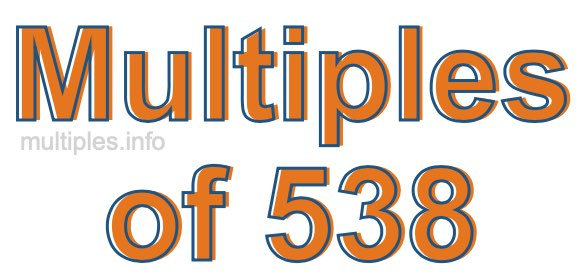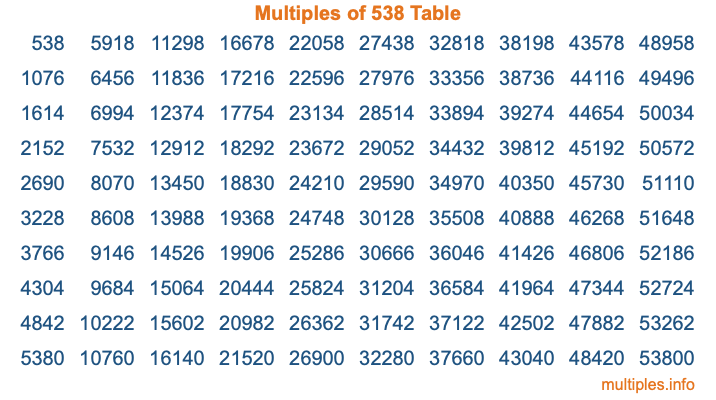Multiples of 538Welcome to the Multiples of 538 page. Here we will first teach you everything you will ever need to know about the multiples of 538, and then give you a study guide summary of everything we taught you to make sure you remember it all. Use this page to look up facts and learn information about the multiples of 538. This page will make you a multiples of five hundred thirty-eight expert!

Definition of Multiples of 538
Multiples of 538 are all the numbers that when divided by 538 equal an integer. Each of the multiples of 538 are called a multiple. A multiple of 538 is created by multiplying 538 by an integer.

Therefore, to create a list of multiples of 538, you start with 1 multiplied by 538, then 2 multiplied by 538, then 3 multiplied by 538, and so on for as long as you want. Thus, the list of the first five multiples of 538 is 538, 1076, 1614, 2152, and 2690. To see a larger list of multiples of 538, see the printable image of Multiples of 538 further down on this page. We also have a category where you can choose any nth multiple of 538.

Multiples of 538 Checker
The Multiples of 538 Checker below checks to see if any number of your choice is a multiple of 538. In other words, it checks to see if there is any number (integer) that when multiplied by 538 will equal your number. To do that, we divide your number by 538. If the the quotient is an integer, then your number is a multiple of 538.

Is  a multiple of 538?

Least Common Multiple of 538 and ...
A Least Common Multiple (LCM) is the lowest multiple that two or more numbers have in common. This is also called the smallest common multiple or lowest common multiple and is useful to know when you are adding our subtracting fractions. Enter one or more numbers below (538 is already entered) to find the LCM.

Check out our LCM Calculator if you need more details about the Least Common Multiple or if you need the LCM for different numbers for adding and subtraction fractions.

nth Multiple of 538
As we stated above, 538 is the first multiple of 538, 1076 is the second multiple of 538, 1614 is the third multiple of 538, and so on. Enter a number below to find the nth multiple of 538.

th multiple of 538

Multiples of 538 vs Factors of 538
538 is a multiple of 538 and a factor of 538, but that is where the similarities end. All postive multiples of 538 are 538 or greater than 538. All positive factors of 538 are 538 or less than 538.

Below is the beginning list of multiples of 538 and the factors of 538 so you can compare:

Multiples of 538: 538, 1076, 1614, 2152, 2690, etc.

Factors of 538: 1, 2, 269, 538

As you can see, the multiples of 538 are all the numbers that you can divide by 538 to get a whole number. The factors of 538, on the other hand, are all the whole numbers that you can multiply by another whole number to get 538.

It's also interesting to note that if a number (x) is a factor of 538, then 538 will also be a multiple of that number (x).

Multiples of 538 vs Divisors of 538
The divisors of 538 are all the integers that 538 can be divided by evenly. Below is a list of the divisors of 538.

Divisors of 538: 1, 2, 269, 538

The interesting thing to note here is that if you take any multiple of 538 and divide it by a divisor of 538, you will see that the quotient is an integer.

Multiples of 538 Table
Below is an image of the first 100 multiples of 538 in a table. The table is in chronological order, column by column. The first column has the first ten multiples of 538, the second column has the next ten multiples of 538, and so on.The Multiples of 538 Table is also referred to as the 538 Times Table or Times Table of 538. You are welcome to print out our table for your studies.

Negative Multiples of 538
Although not often discussed or needed in math, it is worth mentioning that you can make a list of negative multiples of 538 by multiplying 538 by -1, then by -2, then by -3, and so on, to get the following list of negative multiples of 538:

-538, -1076, -1614, -2152, -2690, etc.

Multiples of 538 Summary
Below is a summary of important Multiples of 538 facts that we have discussed on this page. To retain the knowledge on this page, we recommend that you read through the summary and explain to yourself or a study partner why they hold true.

There are an infinite number of multiples of 538.

A multiple of 538 divided by 538 will equal a whole number.

538 divided by a factor of 538 equals a divisor of 538.

The nth multiple of 538 is n times 538.

The largest factor of 538 is equal to the first positive multiple of 538.

538 is a multiple of every factor of 538.

538 is a multiple of 538.

A multiple of 538 divided by a divisor of 538 equals an integer.

538 divided by a divisor of 538 equals a factor of 538.

Any integer times 538 will equal a multiple of 538.

Multiples of a Number
Here you can get the multiples of another number, all with the same attention to detail as we did for multiples of 538 on this page.

Multiples of
Multiples of 539
Did you find our page about multiples of five hundred thirty-eight educational? Do you want more knowledge? Check out the multiples of the next number on our list!